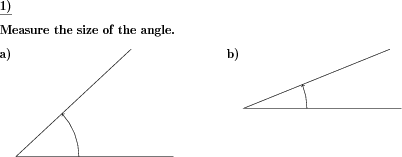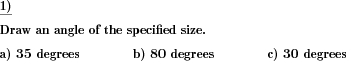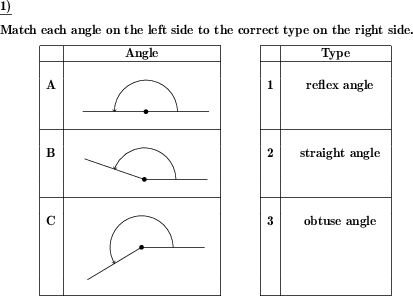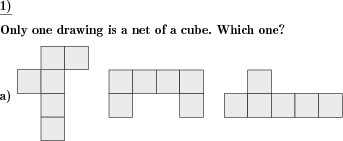Custom math worksheets at your fingertips# Details for problem "Angle types"

Quickname: 6921

Elementary School, Primary School, Junior High School, Middle School, High School.

## Summary

For a given angle, the correct type has to be marked.

## Example## Description

An angle is given in the form of a drawing.

Now, according to its size, the type of this angle has to be determined.

• zero or null angle with a size of 0 degrees.
• acute angle with a size of less than 90 degrees
• right angle with a size of 90 degrees.
• obtuse angle with a size between 90 and 190 degrees.
• straight angle with a size of 180 degrees.
• reflex angle with a size between 180 and 360 degrees.
• full angle with a size of 360 degrees.

The problem is presented in the form of a multiple choice question. Three possibilities are given to choose from. The correct answer has to be marked.

The problem may be configured in a way so that

• all of the types listed may occur,
• that only the five main types exluding full and null/zero angles may occur,
• only the five main types excluding reflex angles may occur.

The length of the angle's rays may be selected from three sizes. The angle size may be customized to be a multiple of five or ten to allow for easier measurement.

The number of problems can be selected.

Download free printable worksheets for this math problem here. The worksheet contains the problems only, the solution sheet includes the answers. Just click on the respective link.

•Worksheet 1Solution sheet with answers
•Worksheet 2Solution sheet with answers
•Worksheet 3Solution sheet with answers

If you can not see the solution sheets for download, they may be filtered out by an ad blocker that you may have installed. If this is the case, please allow ads for this page and reload the page. The solution sheets will then reappear.

These worksheets contain math problems that have to be printed with the correct aspect ratio. When printing, please take care to switch all automatic adjustments, scaling of page, auto adjust, etc, off.

• Do these sample worksheets do not really fit?
• Do you need more math worksheets, with a different level of difficulty?
• Would you like to combine different problems on a worksheet and adjust them to your needs?
• As a teacher, you can put together your own worksheets using the automatically generated math problems provided.
With a free initial credit, you can start creating your own math worksheets in a few minutes.

You can try it for free! Register here, to create custom worksheets now!

## Customization options for this problem

Parameter
Possible values
Number of problems
1, 2, 3, 4
Raster
None, 5 degrees, 10 degrees
Ray length
3cm, 5cm, 7cm
Types
all, not full, null, not reflex,full,null

## Similar problems

Remark
Description
Measure the size of a given angle
As a matching task - angles and types given
Given angles have to be matched to given types

## dw-Math worksheet templates that do contain this problem

Name
Title
Description
Angles and angle types
Measure and draw angles, identify types of angles

## Other types of problems that appear on worksheets with this problem:

Relevance
Name
Description
Quickname
Example
****
Angle size measurement
Measure the size of a given angle****
Angle to be drawn
An angle of a given size has to be drawn.**
Match angles to angle types
Given angles have to be matched to given types**
Identify cube nets in a series of cube-like nets
A series of drawings is shown. Which ones represent cube nets?Deutsche Version dieser Aufgabe
These informational pages with samples describe math problems that can be combined on custom math worksheets with solutions for home and K-12 school use.
Deutsche Seiten
×